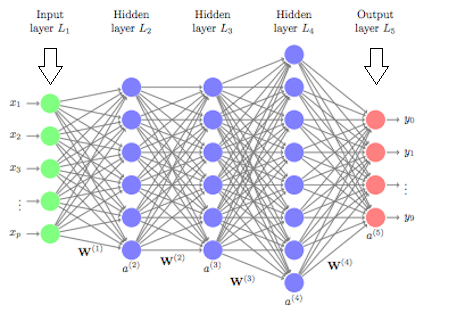# Deep Learning for Air Quality Prediction

Original article was published by Ashan Lakmal on Deep Learning on MediumNow From the above part, we can go through some idea of the LSTM neural networks. So next move on to the Coding part. Here we choose python as the programming languages using the following libraries.

`import datetime, warnings, scipy import pandas as pdimport numpy as npimport seaborn as snsimport matplotlib as mplimport matplotlib.pyplot as pltfrom IPython.core.interactiveshell import InteractiveShellimport mathfrom keras.models import Sequentialfrom keras.layers import Densefrom keras.layers import LSTMfrom sklearn.preprocessing import MinMaxScaler, StandardScalerfrom sklearn.metrics import mean_squared_error`

After importing the libraries then move to the data importing a part of the algorithm.

`df = pd.read_csv('Data.csv', sep=' ')`

So after importing the data the main process of the algorithm comes next. The calculation part for the quantile from each attribute. The following code snippet tells how the calculation was done.

`def calculate_quantile (i, df2):    Q1 = df2[[i]].quantile(0.25)    Q3 = df2[[i]].quantile(0.75)    IQR = Q3 - Q1    min = df2[[i]].min()    max = df2[[i]].max()    min_IQR = Q1 - 1.5*IQR    max_IQR = Q3 + 1.5*IQR    return Q1, Q3, min, max, min_IQR, max_IQR# delete first and last rows to avoid missing value extrapolationdf2.drop(index=[df2.index, df2.index[df2.shape-1]], inplace=True)# find and interpolate the outliersfor i in df2.columns:    print('\nAttribute-',i,':')    Q1, Q3, min, max, min_IQR, max_IQR = calculate_quantile(i, df2)    print('Q1 = %.2f' % Q1)    print('Q3 = %.2f' % Q3)    print('min IQR = %.2f' % min_IQR)    print('max IQR = %.2f' % max_IQR)    if (min < min_IQR):        print('Low outlier = %.2f' % min)    if (max > max_IQR):        print('High outlier= %.2f' % max)    def convert_nan (x, max_IQR=max_IQR, min_IQR=min_IQR):        if ((x > max_IQR) | (x < min_IQR)):            x = np.nan        else:            x = x        return xdef convert_nan_HUM (x, max_IQR=100.0, min_IQR=min_IQR):        if ((x > max_IQR) | (x < min_IQR)):            x = np.nan        else:            x = x        return x    if (i == 'HUM'):        df2[i] = df2[i].map(convert_nan_HUM)        df2[i] = df2[i].interpolate(method='linear')    if (i != 'HUM'):        df2[i] = df2[i].map(convert_nan)        df2[i] = df2[i].interpolate(method='linear')    if (len(df2[df2[i].isnull()][i]) == 0):`

After the calculation then move to the next part which most important part of here the prediction part. So for this step, the data will split into some stages. Here we split the data 75% for training and 25% for testing.

`train_size = int(len(dataset) * 0.75)test_size = len(dataset) - train_sizetrain, test = dataset[0:train_size,:], dataset[train_size:len(dataset),:]print(len(train), len(test))`

After doing that, Now the LSTM neural network will create. Following is how the LSTM neural network was made.

`model = Sequential()model.add(LSTM(4, input_shape=(1, look_back)))model.add(Dense(1))model.compile(loss='mean_squared_error', optimizer='adam')model.fit(trainX, trainY, epochs=1500, batch_size=32, verbose=2)`

After creating a neural network all the prediction work is done. Now the results will tell how good was the prediction. Here is some example that i have done to analyze the temperature.

`# shift train predictions for plottingtrainPredictPlot = np.empty_like(dataset)trainPredictPlot[:, :] = np.nantrainPredictPlot[look_back:len(trainPredict)+look_back, :] = trainPredict# shift test predictions for plottingtestPredictPlot = np.empty_like(dataset)testPredictPlot[:, :] = np.nantestPredictPlot[len(trainPredict)+(look_back*2)+1:len(dataset)-1, :] = testPredict# plot original dataset and predictionstime_axis = np.linspace(0, dataset.shape-1, 15)time_axis = np.array([int(i) for i in time_axis])time_axisLab = np.array(df2.index, dtype='datetime64[D]')fig = plt.figure()ax = fig.add_axes([0, 0, 2.1, 2])ax.plot(np.expm1(dataset), label='Original Dataset')ax.plot(trainPredictPlot, color='orange', label='Train Prediction')ax.plot(testPredictPlot, color='red', label='Test Prediction')ax.set_xticks(time_axis)ax.set_xticklabels(time_axisLab[time_axis], rotation=45)ax.set_xlabel('\nDate', fontsize=27, fontweight='bold')ax.set_ylabel('TEMP', fontsize=27, fontweight='bold')ax.legend(loc='best', prop= {'size':20})ax.tick_params(size=10, labelsize=15)ax.set_xlim([-1,1735])ax1 = fig.add_axes([2.3, 1.3, 1, 0.7])ax1.plot(np.expm1(dataset), label='Original Dataset')ax1.plot(testPredictPlot, color='red', label='Test Prediction')ax1.set_xticks(time_axis)ax1.set_xticklabels(time_axisLab[time_axis], rotation=45)ax1.set_xlabel('Date', fontsize=27, fontweight='bold')ax1.set_ylabel('TEMP', fontsize=27, fontweight='bold')ax1.tick_params(size=10, labelsize=15)ax1.set_xlim([1360,1735]);`

Here is the output of it